# High School Math : Proportionalities

## Example Questions

### Example Question #1 : Basic Single Variable Algebra

Sarah notices her map has a scale of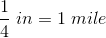.  She measures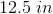between Beaver Falls and Chipmonk Cove.  How far apart are the cities?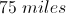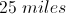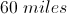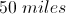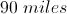Explanation:is the same as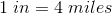So to find out the distance between the cities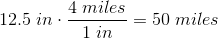### Example Question #2 : Basic Single Variable Algebra

Sunshine paint is made by mixing three parts yellow paint and one part red paint. How many gallons of yellow paint should be mixed with two quarts of red paint?

(1 gallon = 4 quarts)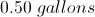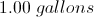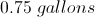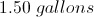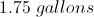Explanation:

First set up the proportion: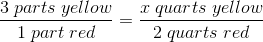x =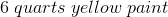Then convert this to gallons: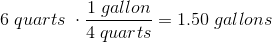### Example Question #1 : Understanding Direct Proportionality

Ifis directly proportional to, andwhen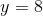, find a formula for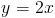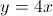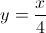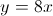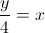Explanation: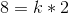sinceandSolving for, you get that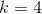Replacingin the original equation, you get the answer of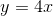### Example Question #1 : Understanding Indirect Proportionality

Ifis inversely proportional to, and ifwhen, find a formula for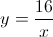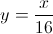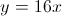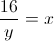Since y is inversely porportional to x, you can use the standard equations of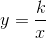where k is a constant. Plugging the given x and y into this equation to solve for k,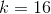. To get the answer, substitute k into the standard equation.